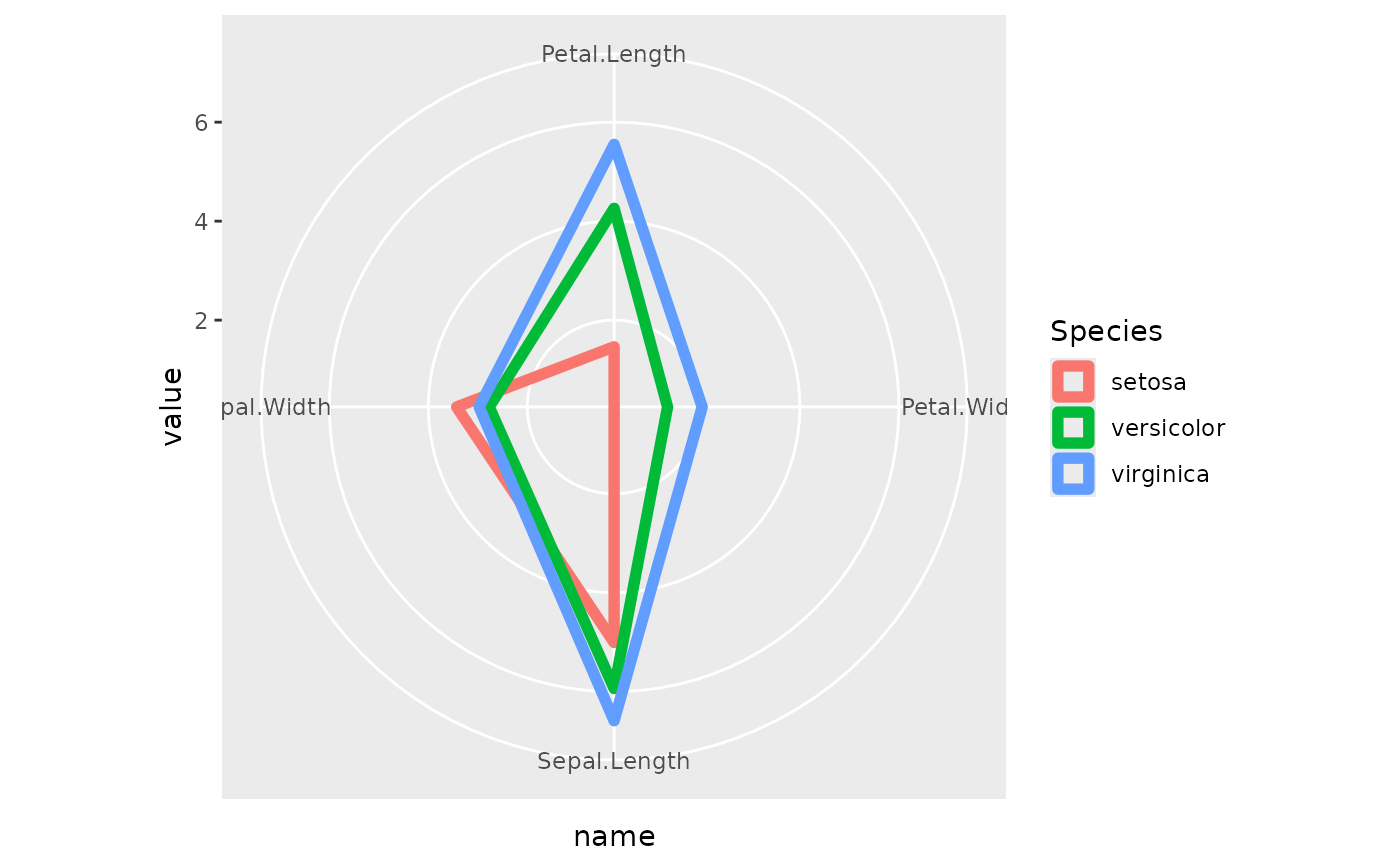## Usage

coord_radar(theta = "x", start = 0, direction = 1, ...)

## Arguments

theta

variable to map angle to (x or y)

start

Offset of starting point from 12 o'clock in radians. Offset is applied clockwise or anticlockwise depending on value of direction.

direction

1, clockwise; -1, anticlockwise

...

Other arguments to be passed to ggproto.

## Examples

# Create a radar/spider chart with ggplot:
if (require("poorman") && require("ggplot2")) {
data <- iris[-5] %>%
aggregate(list(Species = iris\$Species), mean) %>%
datawizard::reshape_longer(2:5)

data %>%
ggplot(aes(x = Name, y = Value, color = Species, group = Species)) +
geom_polygon(fill = NA, size = 2) +